## BM 算法的核心思想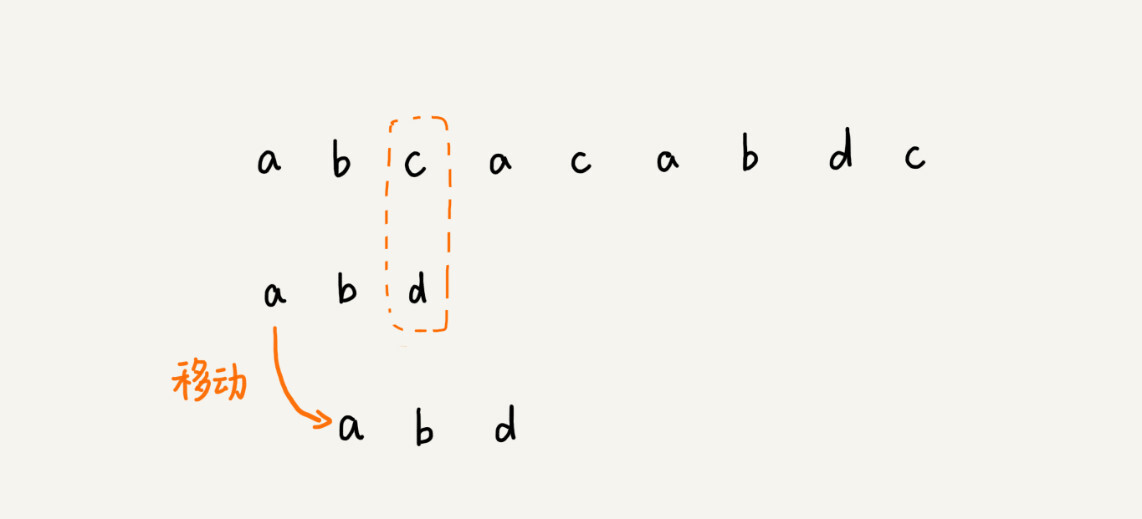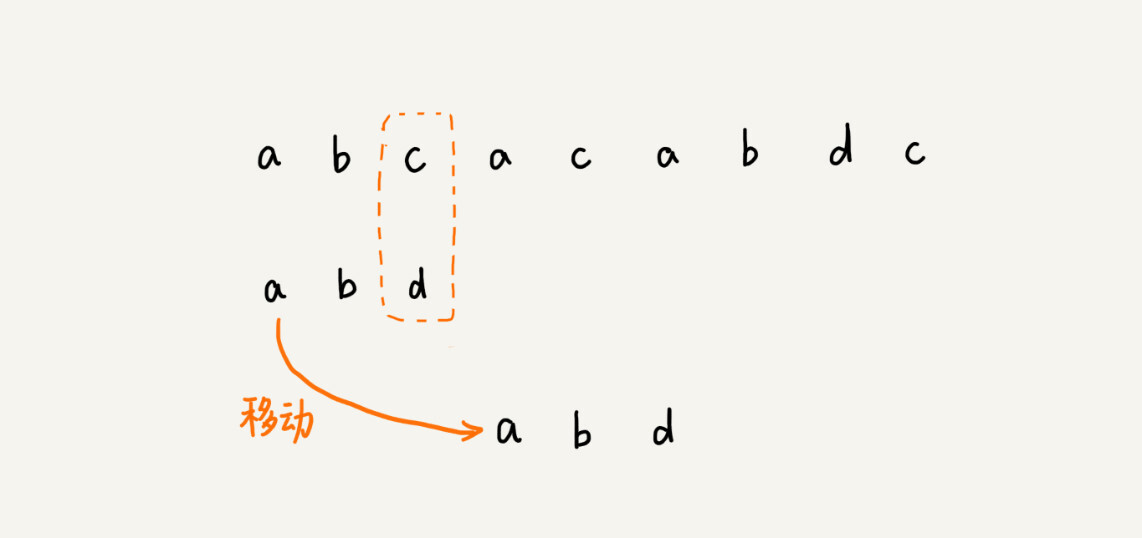## BM 算法原理分析

BM 算法包含两部分，分别是坏字符规则bad character rule）和好后缀规则good suffix shift）。我们下面依次来看，这两个规则分别都是怎么工作的。

### 1. 坏字符规则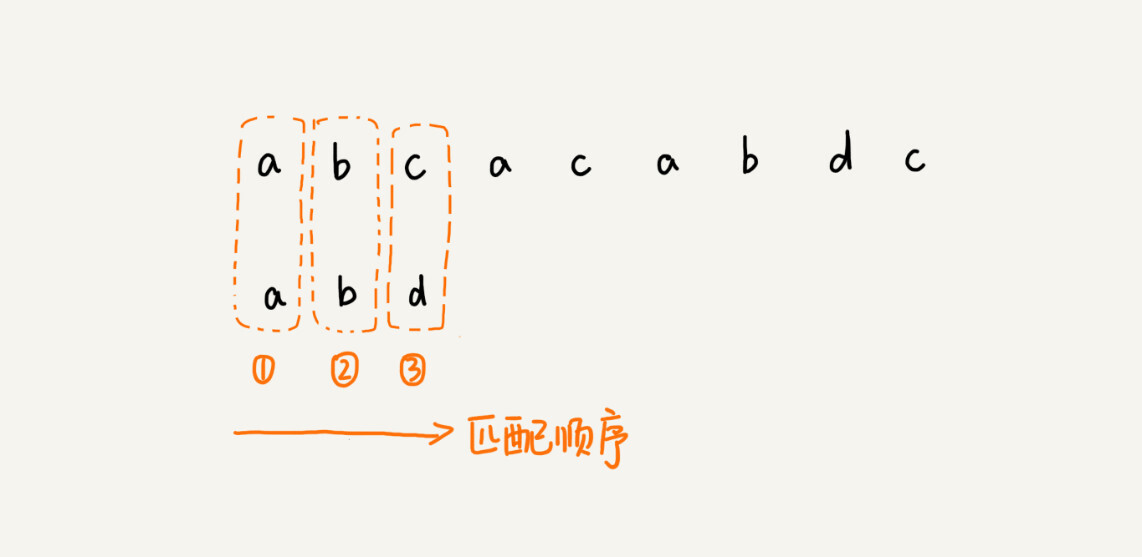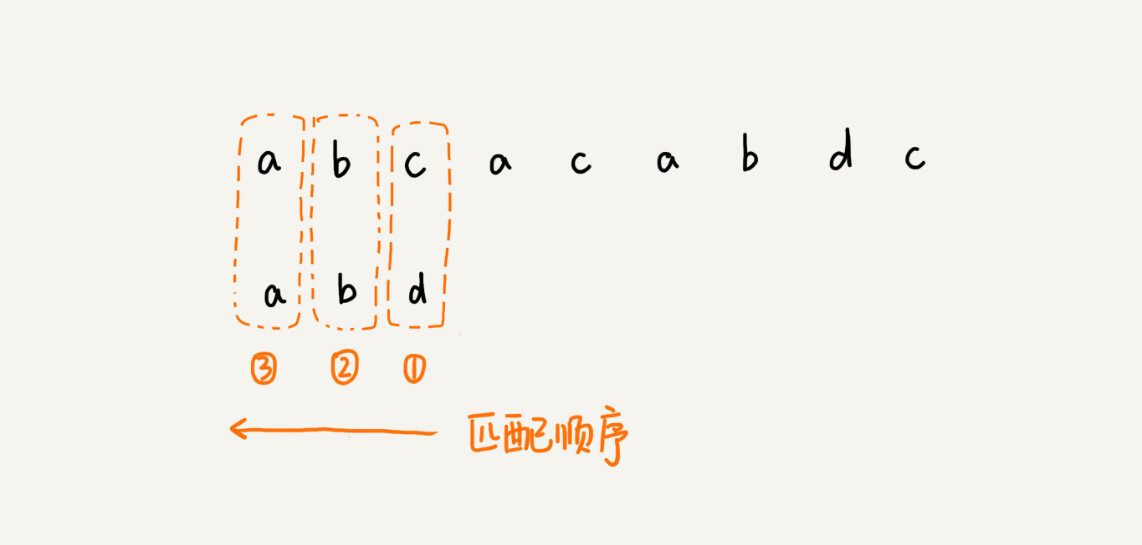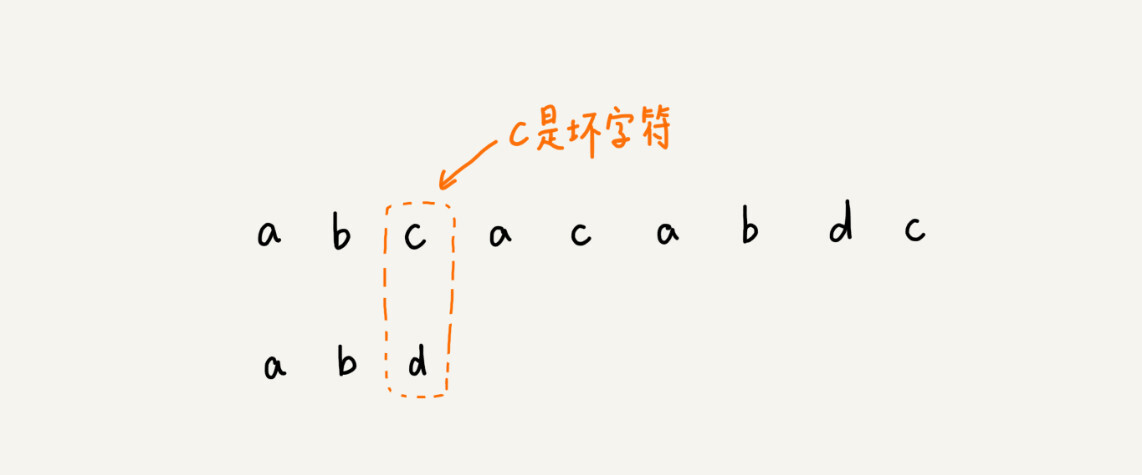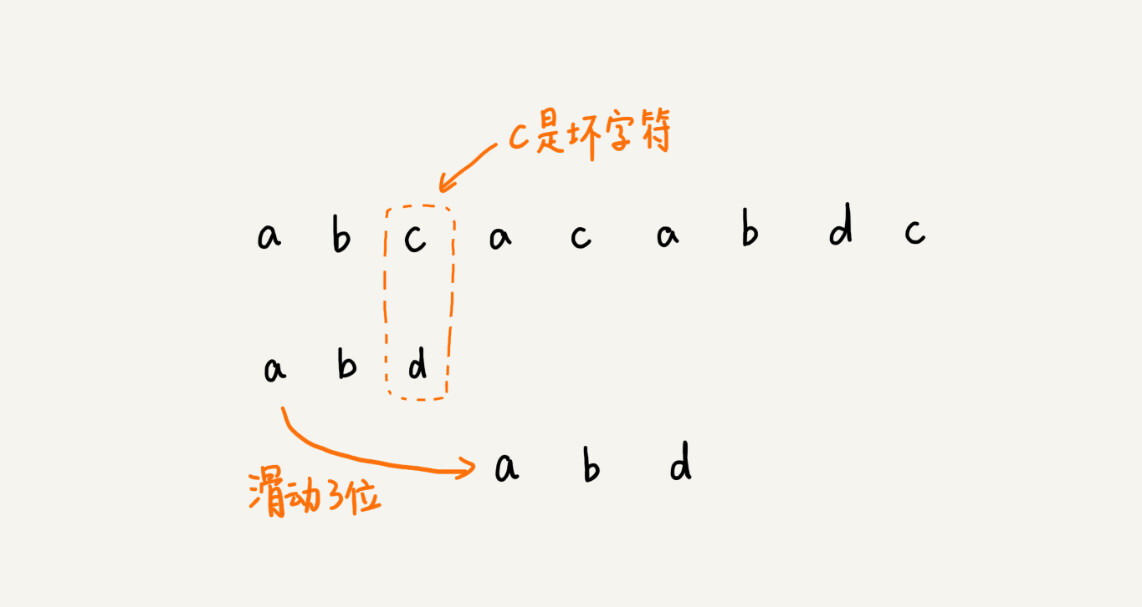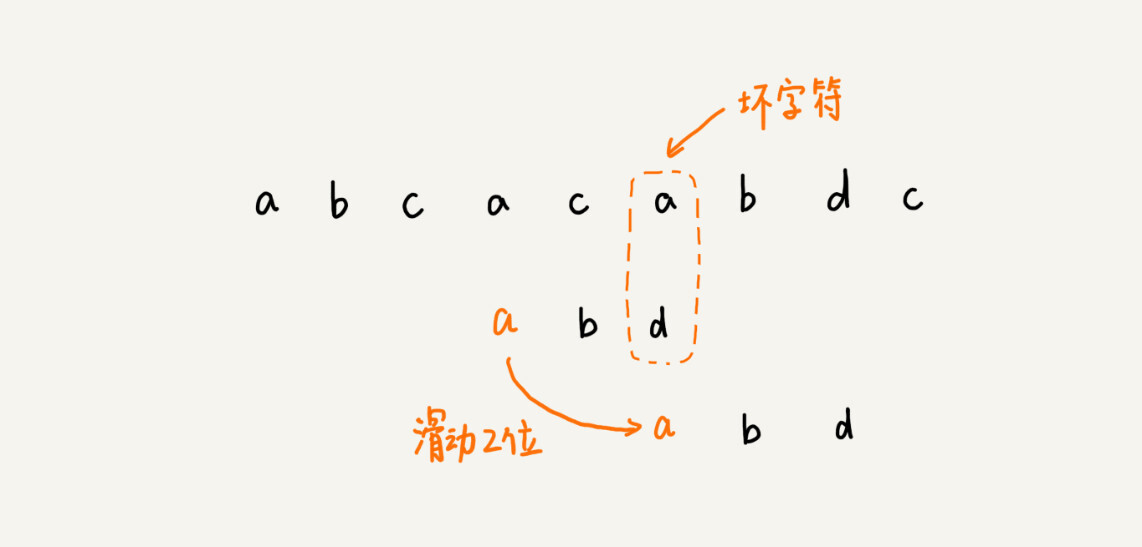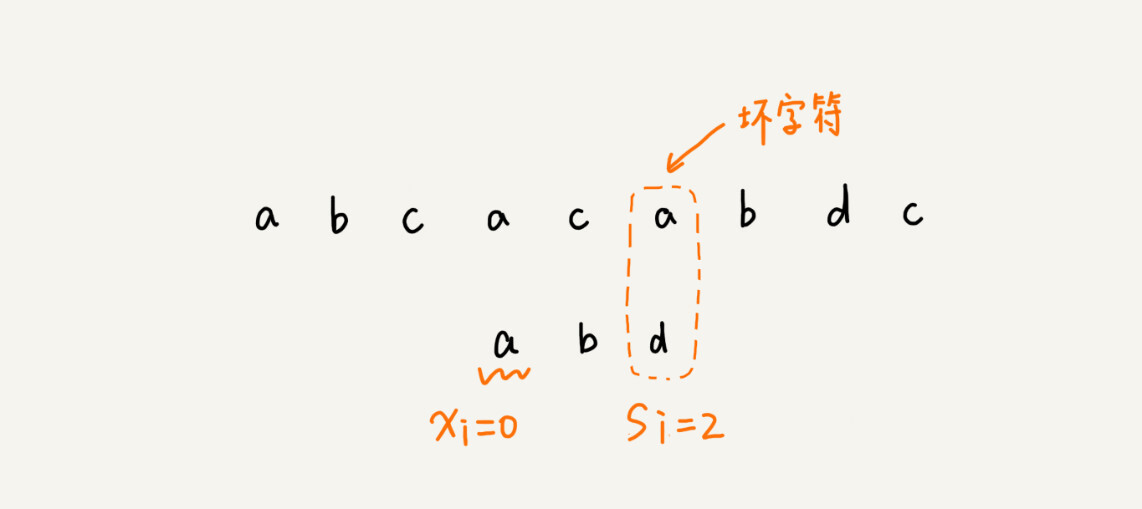### 2. 好后缀规则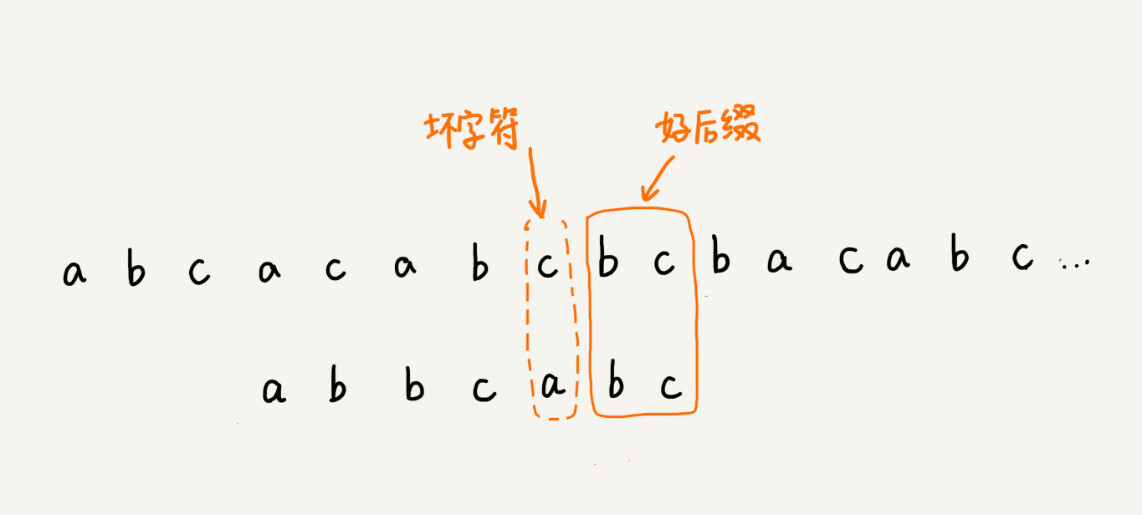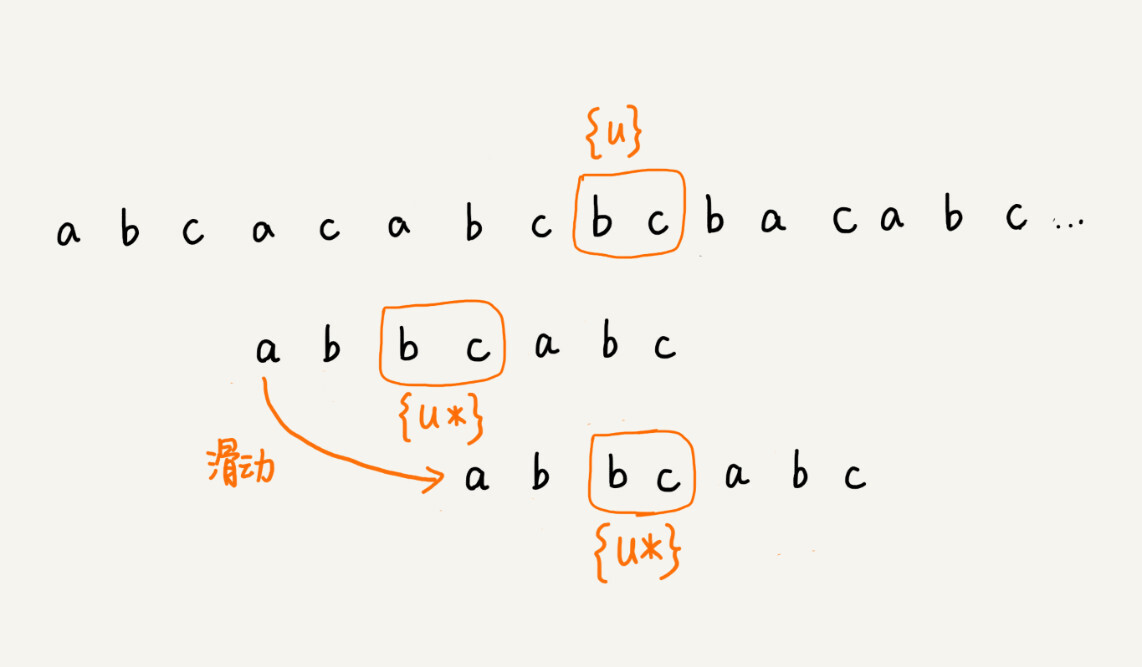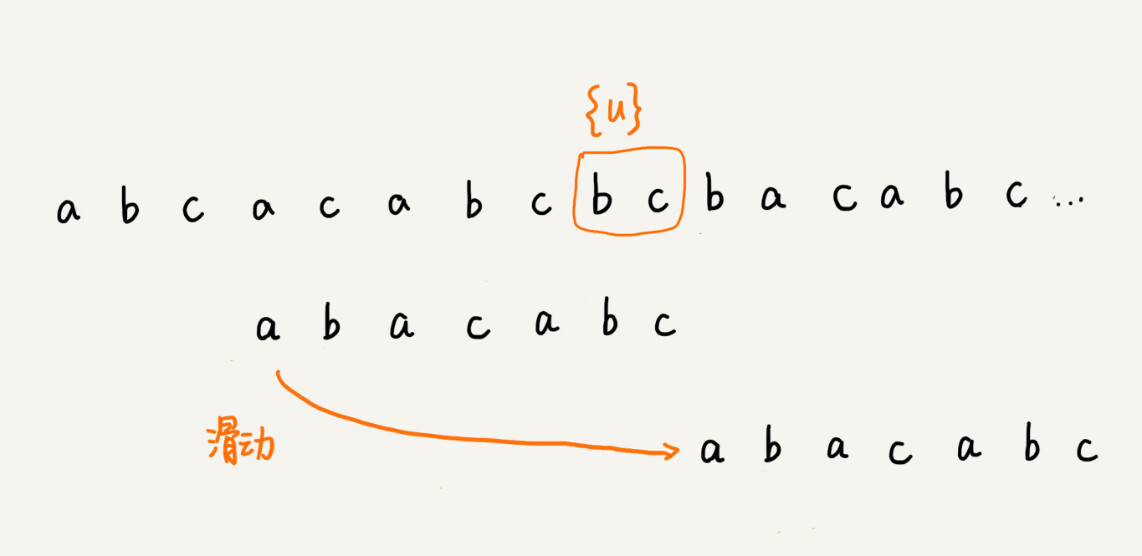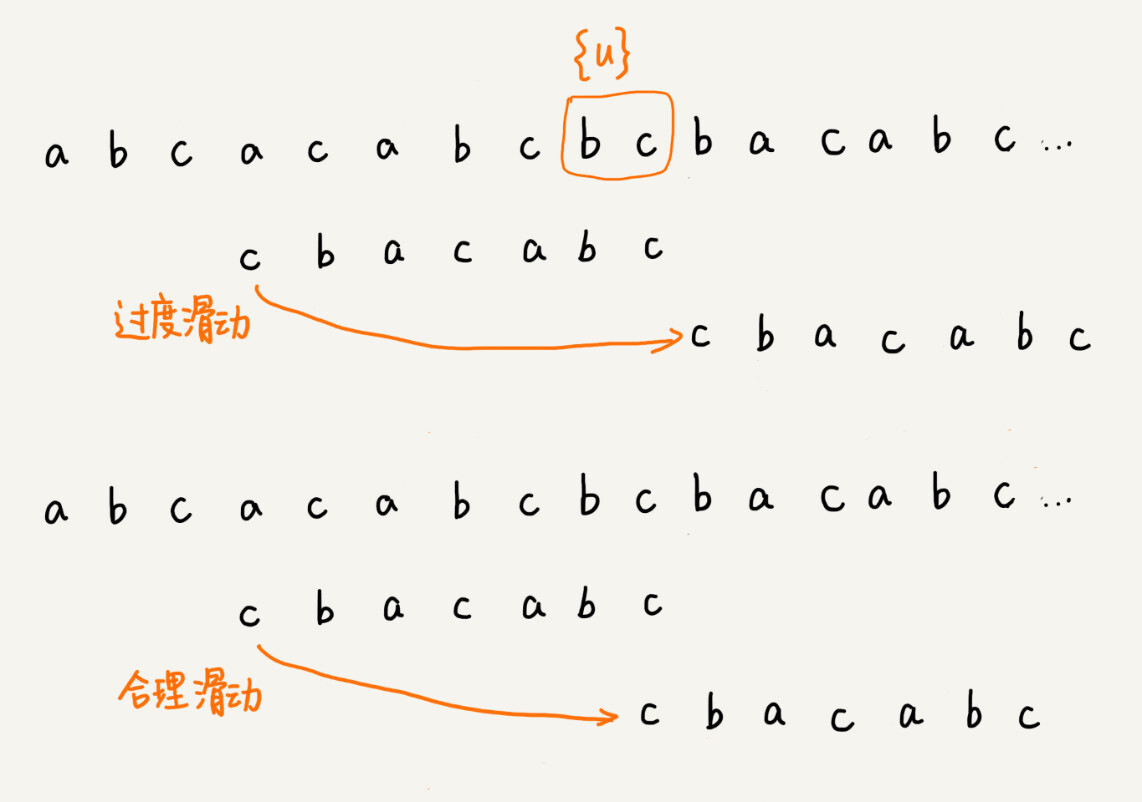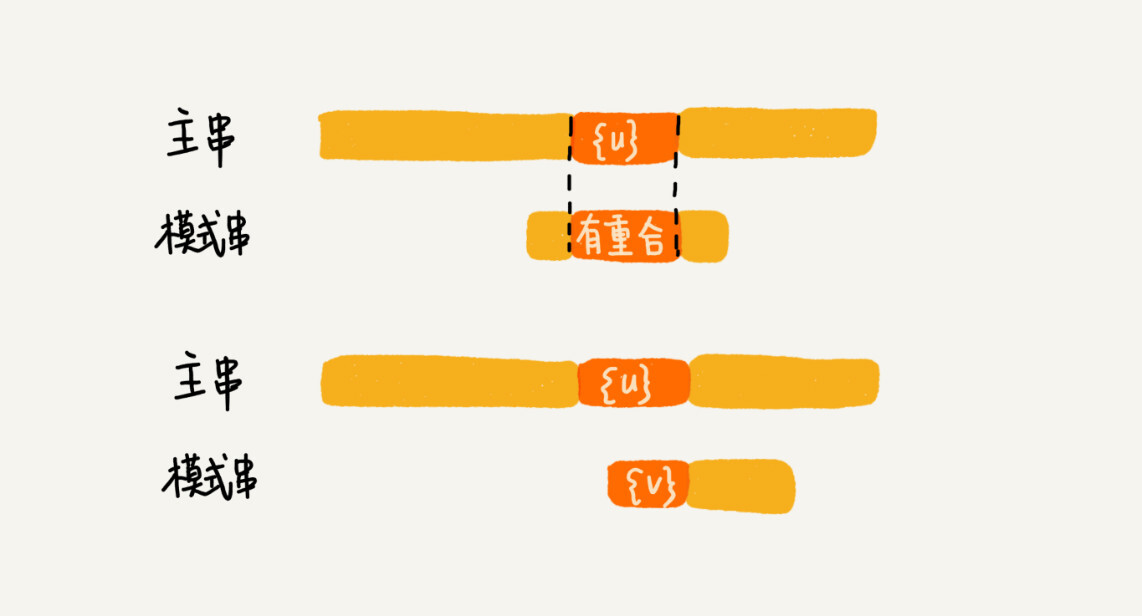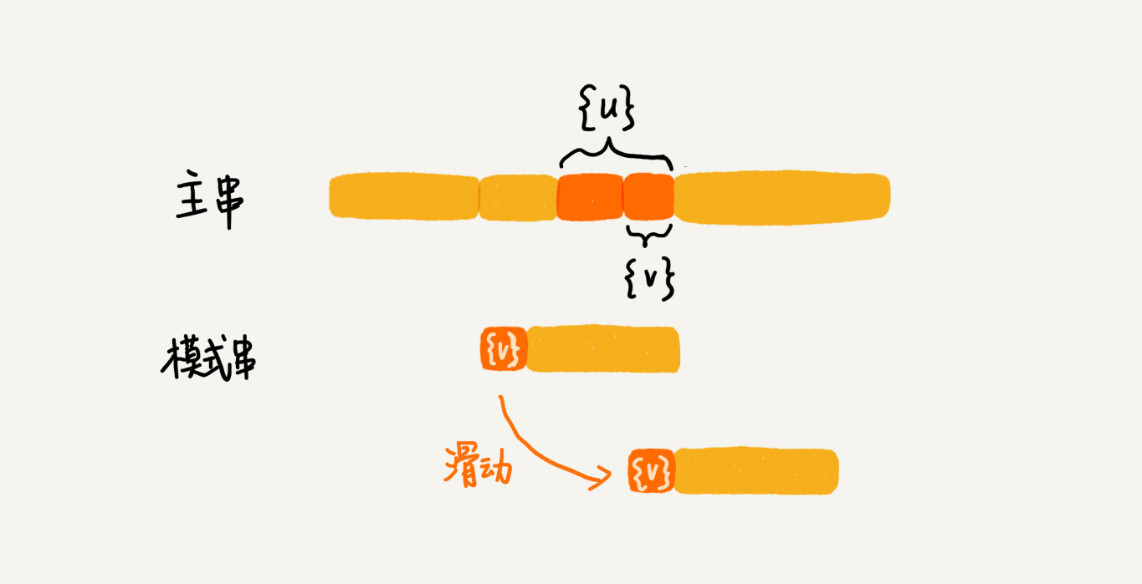## BM 算法代码实现

“坏字符规则”本身不难理解。当遇到坏字符时，要计算往后移动的位数 si-xi，其中 xi 的计算是重点，我们如何求得 xi 呢？或者说，如何查找坏字符在模式串中出现的位置呢？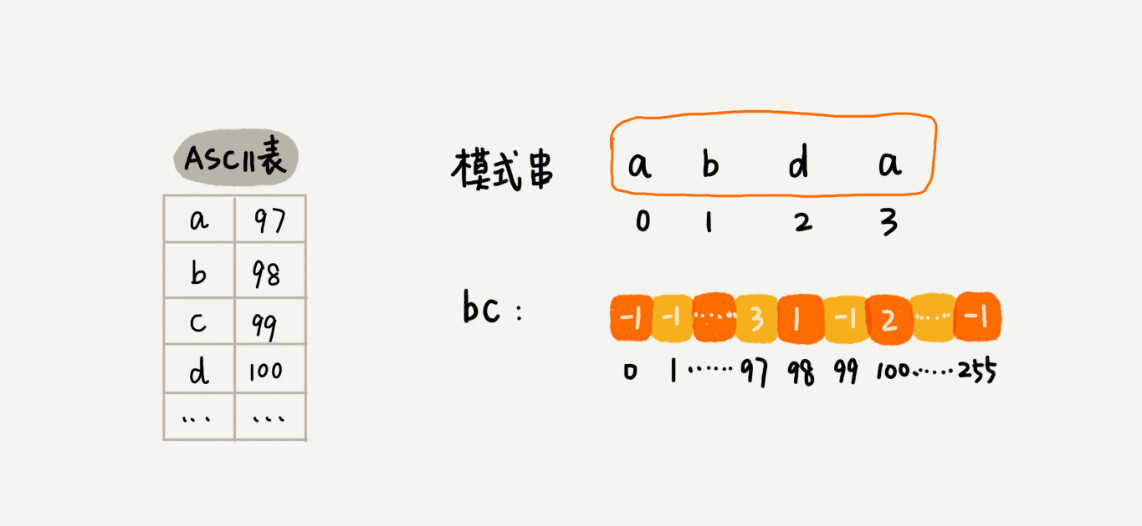```private static final int SIZE = 256; // 全局变量或成员变量
private void generateBC(char[] b, int m, int[] bc) {
for (int i = 0; i < SIZE; ++i) {
bc[i] = -1; // 初始化bc
}
for (int i = 0; i < m; ++i) {
int ascii = (int)b[i]; // 计算b[i]的ASCII值
bc[ascii] = i;
}
}```

```public int bm(char[] a, int n, char[] b, int m) {
int[] bc = new int[SIZE]; // 记录模式串中每个字符最后出现的位置
generateBC(b, m, bc); // 构建坏字符哈希表
int i = 0; // i表示主串与模式串对齐的第一个字符
while (i <= n - m) {
int j;
for (j = m - 1; j >= 0; --j) { // 模式串从后往前匹配
if (a[i+j] != b[j]) break; // 坏字符对应模式串中的下标是j
}
if (j < 0) {
return i; // 匹配成功，返回主串与模式串第一个匹配的字符的位置
}
// 这里等同于将模式串往后滑动j-bc[(int)a[i+j]]位
i = i + (j - bc[(int)a[i+j]]);
}
return -1;
}```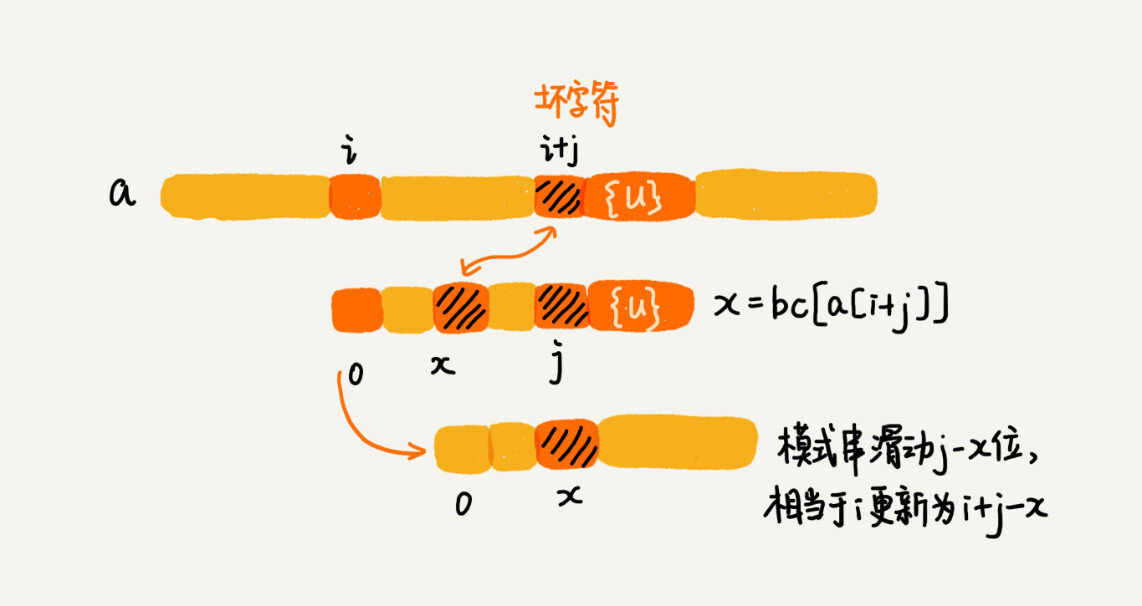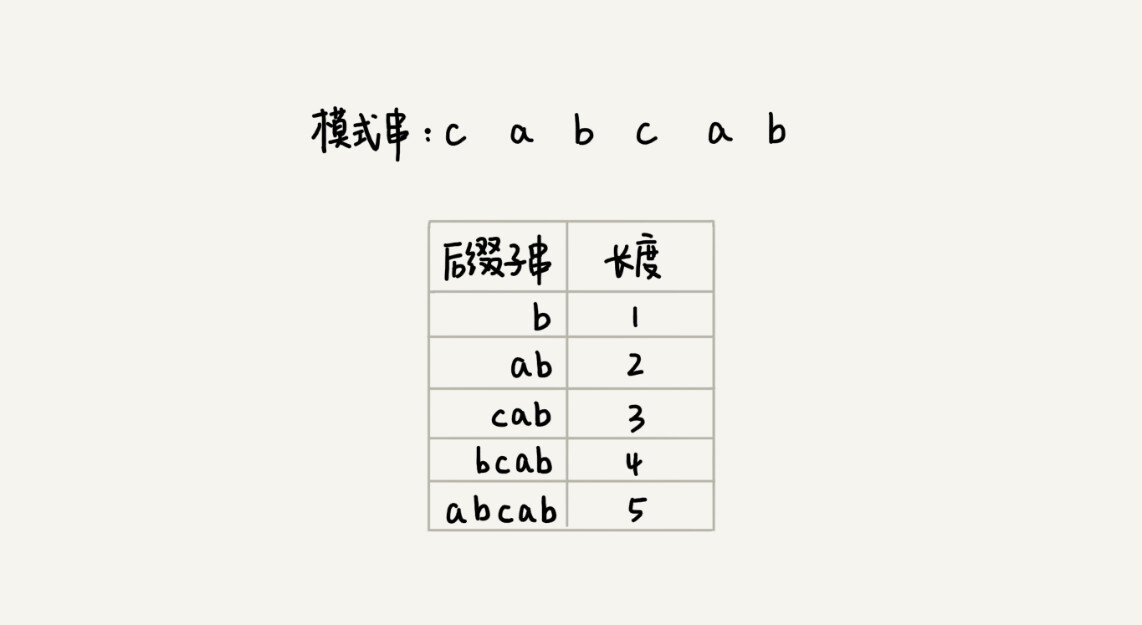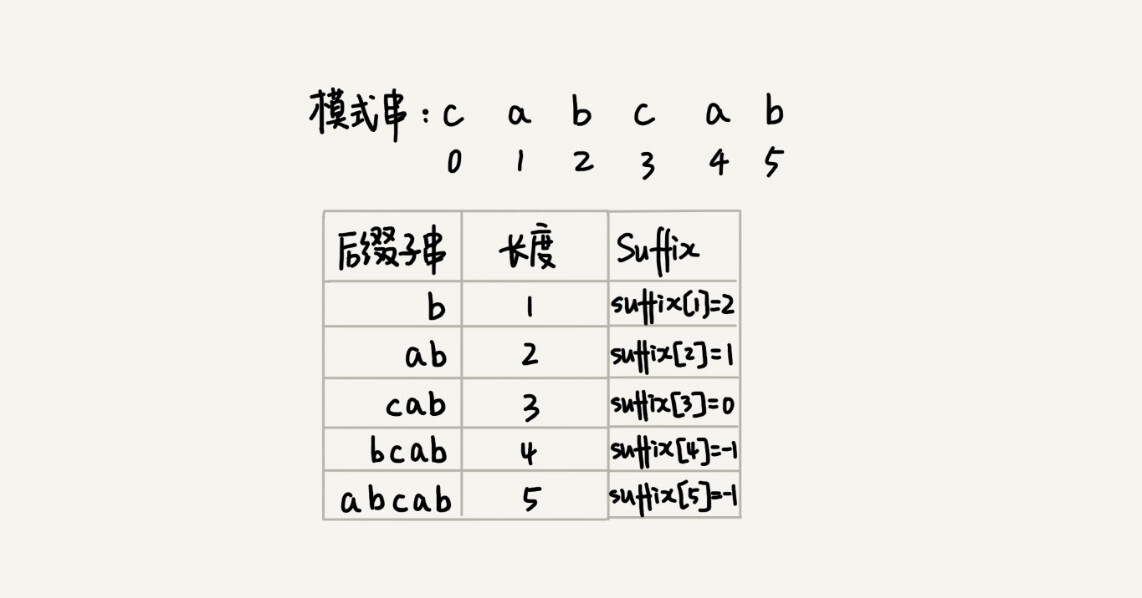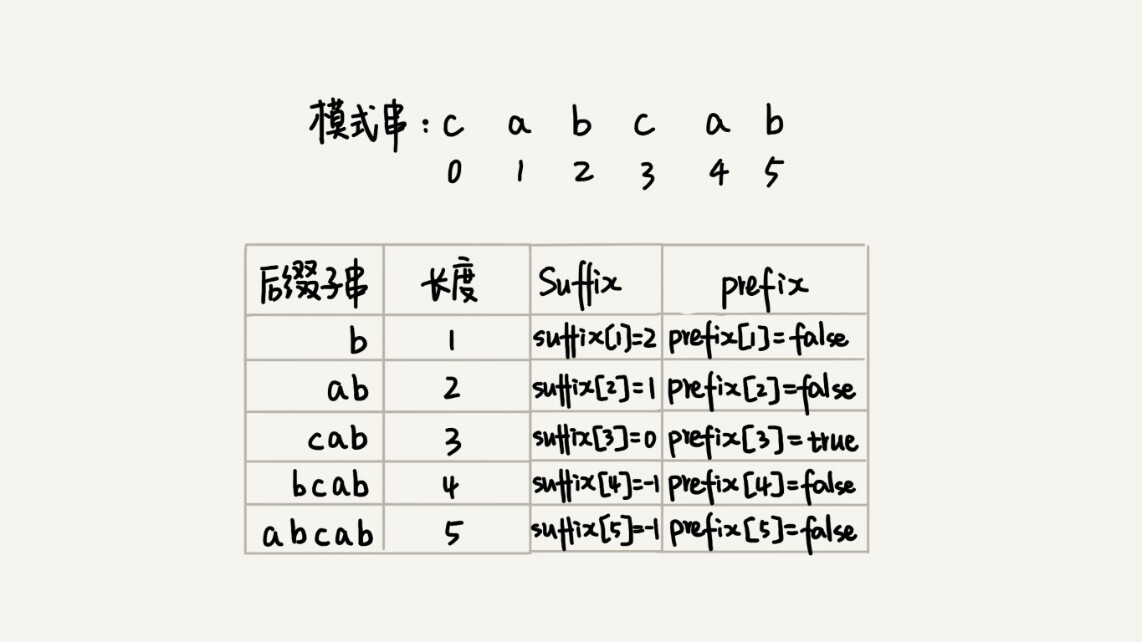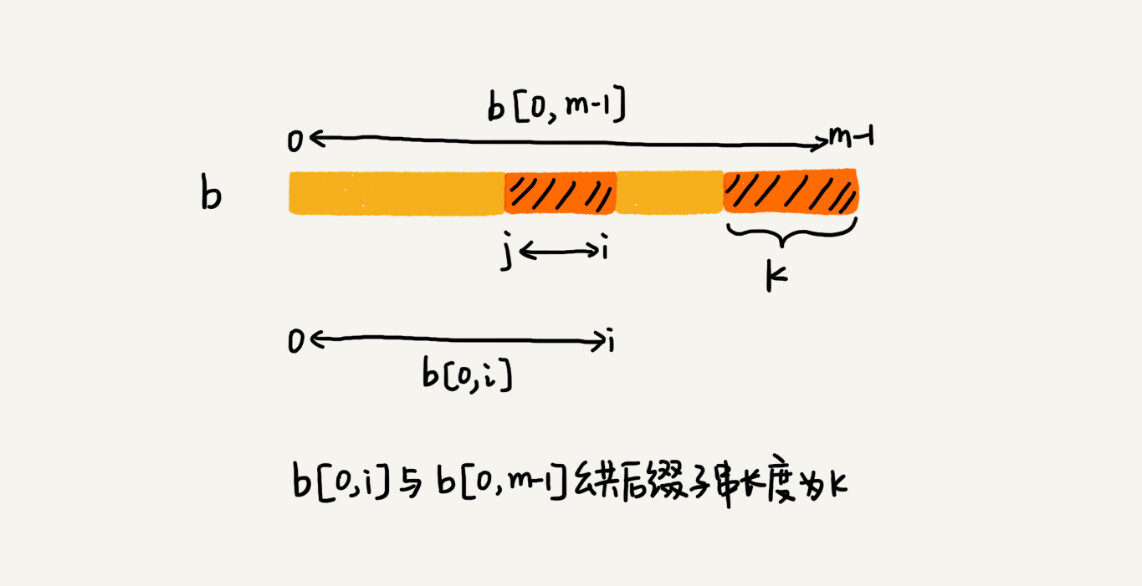```// b表示模式串，m表示长度，suffix，prefix数组事先申请好了
private void generateGS(char[] b, int m, int[] suffix, boolean[] prefix) {
for (int i = 0; i < m; ++i) { // 初始化
suffix[i] = -1;
prefix[i] = false;
}
for (int i = 0; i < m - 1; ++i) { // b[0, i]
int j = i;
int k = 0; // 公共后缀子串长度
while (j >= 0 && b[j] == b[m-1-k]) { // 与b[0, m-1]求公共后缀子串
--j;
++k;
suffix[k] = j+1; //j+1表示公共后缀子串在b[0, i]中的起始下标
}
if (j == -1) prefix[k] = true; //如果公共后缀子串也是模式串的前缀子串
}
}```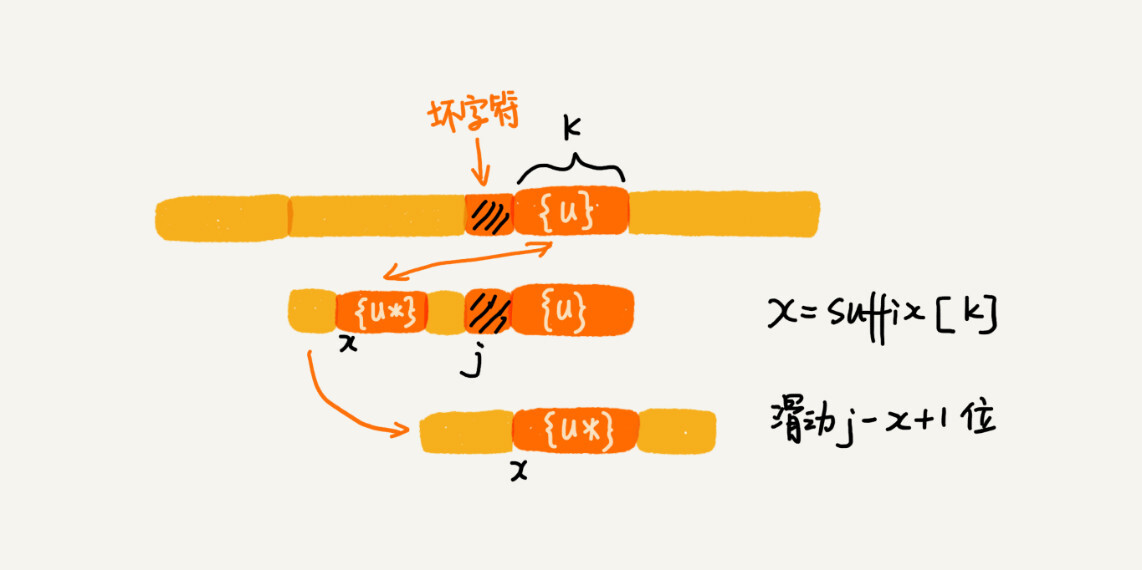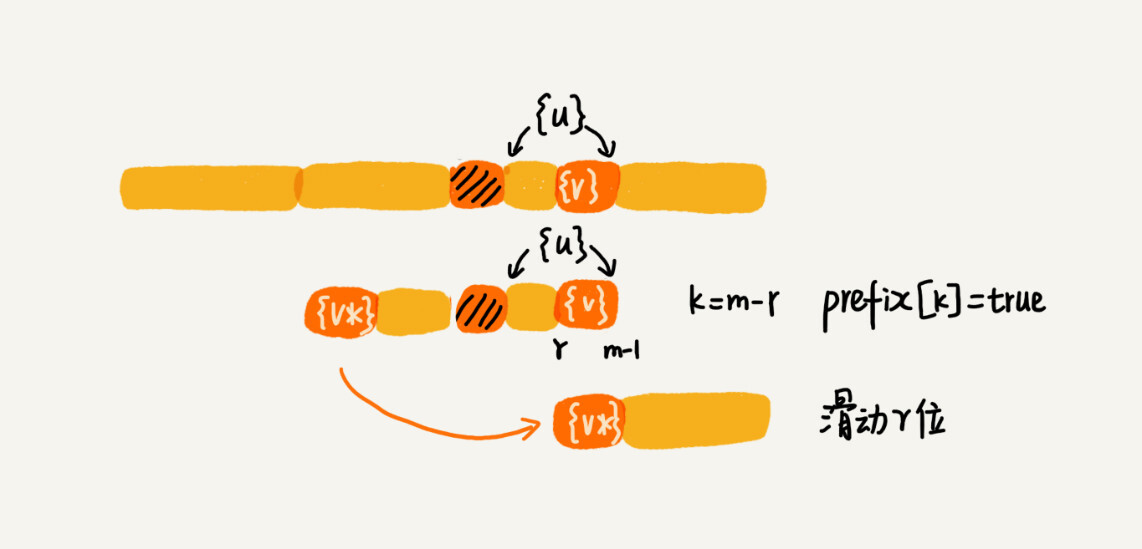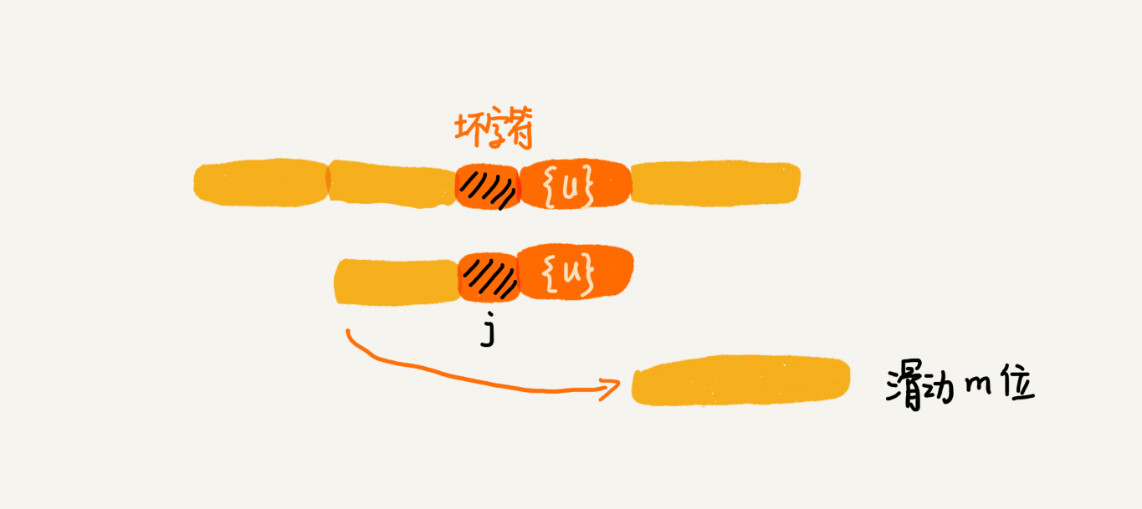```// a,b表示主串和模式串；n，m表示主串和模式串的长度。
public int bm(char[] a, int n, char[] b, int m) {
int[] bc = new int[SIZE]; // 记录模式串中每个字符最后出现的位置
generateBC(b, m, bc); // 构建坏字符哈希表
int[] suffix = new int[m];
boolean[] prefix = new boolean[m];
generateGS(b, m, suffix, prefix);
int i = 0; // j表示主串与模式串匹配的第一个字符
while (i <= n - m) {
int j;
for (j = m - 1; j >= 0; --j) { // 模式串从后往前匹配
if (a[i+j] != b[j]) break; // 坏字符对应模式串中的下标是j
}
if (j < 0) {
return i; // 匹配成功，返回主串与模式串第一个匹配的字符的位置
}
int x = j - bc[(int)a[i+j]];
int y = 0;
if (j < m-1) { // 如果有好后缀的话
y = moveByGS(j, m, suffix, prefix);
}
i = i + Math.max(x, y);
}
return -1;
}

// j表示坏字符对应的模式串中的字符下标; m表示模式串长度
private int moveByGS(int j, int m, int[] suffix, boolean[] prefix) {
int k = m - 1 - j; // 好后缀长度
if (suffix[k] != -1) return j - suffix[k] +1;
for (int r = j+2; r <= m-1; ++r) {
if (prefix[m-r] == true) {
return r;
}
}
return m;
}```

## 解答开篇 & 内容小结

BM 算法核心思想是，利用模式串本身的特点，在模式串中某个字符与主串不能匹配的时候，将模式串往后多滑动几位，以此来减少不必要的字符比较，提高匹配的效率。BM 算法构建的规则有两类，坏字符规则和好后缀规则。好后缀规则可以独立于坏字符规则使用。因为坏字符规则的实现比较耗内存，为了节省内存，我们可以只用好后缀规则来实现 BM 算法。